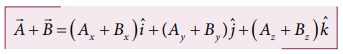Home | | Physics 11th std | Vector addition using components

## Chapter: 11th Physics : UNIT 2 : Kinematics

the addition and subtraction of vectors becomes much easier to perform.

In the previous section we have learnt about addition and subtraction of two vectors using geometric methods. But once we choose a coordinate system, the addition and subtraction of vectors becomes much easier to perform.

The two vectorsandin a Cartesian coordinate system can be expressed asThen the addition of two vectors is equivalent to adding their corresponding x, y and z components.Similarly the subtraction of two vectors is equivalent to subtracting the corresponding x, y and z components.The above rules form an analytical way of adding and subtracting two vectors.Tags : with Solved Example Problems , 11th Physics : UNIT 2 : Kinematics
Study Material, Lecturing Notes, Assignment, Reference, Wiki description explanation, brief detail
11th Physics : UNIT 2 : Kinematics : Vector addition using components | with Solved Example Problems